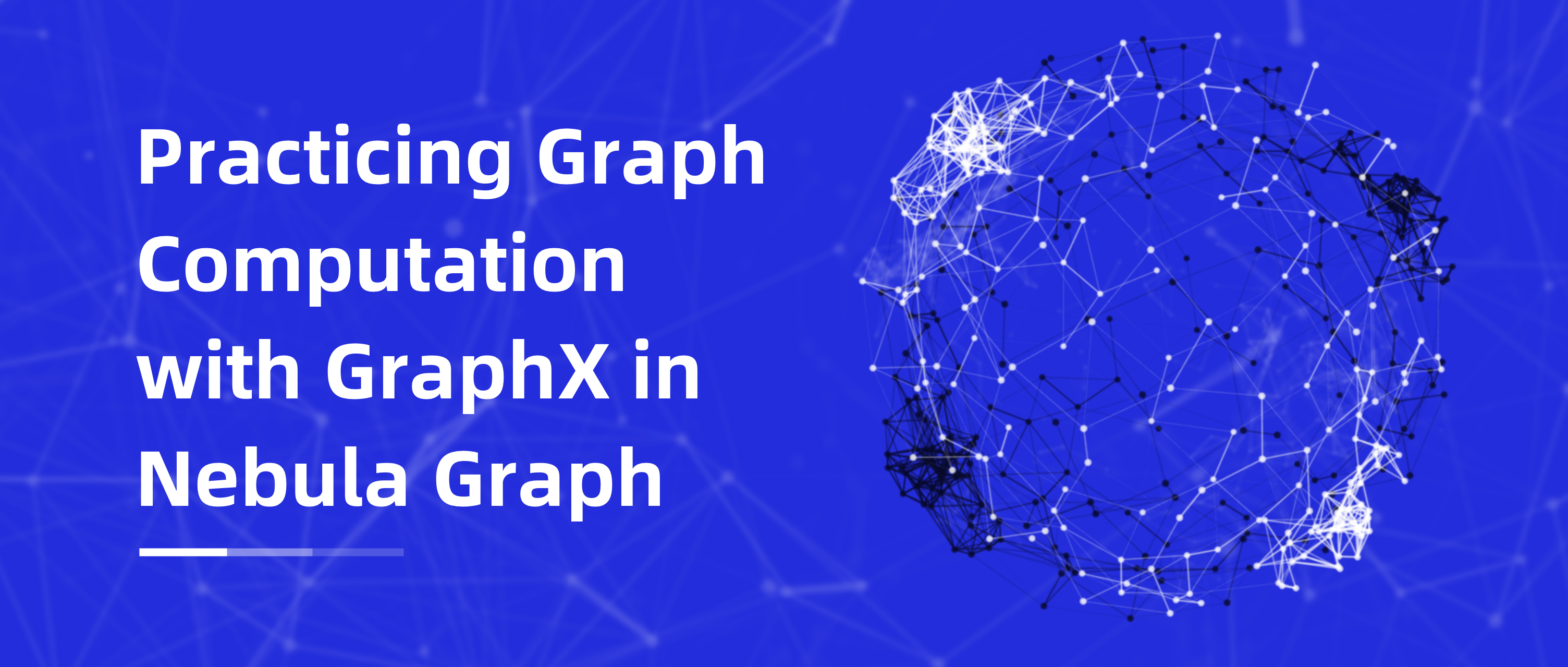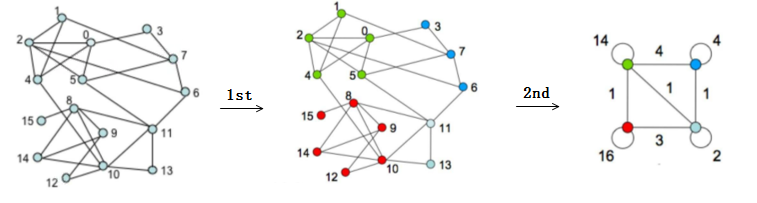# Practicing Graph Computation with GraphX in Nebula GraphNicole Wang2020-11-19featuresWith the rapid development of network information technology, data is gradually developing towards multi-source heterogeneity. Inside the multi-source heterogeneous data lies countless inextricable relations. And this kind of relations, together with the the network structure, are sure essential for data analysis. Unfortunately, when it comes to large scale data analysis, the traditional relational databases are poor in association detection and expressions. Therefore, graph data has attracted great attention for its powerful ability in expressions. Graph computing uses a graph model to express and solve the problem. Graphs can integrate with multi-source data types. In addition to displaying the static basic features for data, graph computing also finds its chance to display the graph structure and relationships hidden in the data. Thus graph becomes an important analysis tool in social network, recommendation system, knowledge graph, financial risk control, network security and text retrieval.

## Practical Algorithms

In order to support the business needs of large-scale graph computing, Nebula Graph provides PageRank and Louvain community-discovered graph computing algorithms based on GraphX. Users can execute these algorithm applications by submitting Spark tasks. In addition, users can also write Spark programs by using Spark Connector to call other built-in graph algorithms in GraphX, such as LabelPropagation, ConnectedComponent, etc.

### PageRank

PageRank is an algorithm raised by Google to rank web pages in their search engine results. PageRank is a way of measuring the importance of website pages.

#### Introduction to PageRank

PageRank was named after Larry Page and Sergey Brin from Stanford in the United States. PageRank works by counting the number and quality of links to a page to determine a rough estimate of how important the website is. The underlying assumption is that more important websites are likely to receive more links from other websites.

#### Applications of PageRank

##### Content recommendation based on similarity

With the help of the PageRank, you can recommend similar content to users based on their browse history and view time when analyzing social applications such as Twitter and Facebook.

##### Analyze the social influence of users

You can analyze the social influence of users according to their PageRank values in social network analysis.

##### Importance research for papers

Judge a paper’s quality according to its PageRank value. Actually the PageRank algorithm comes from the idea of judging the quality of the papers. In addition, PageRank also finds its usage in data analysis and mining.

#### Implementation PageRank

PageRank in GraphX is implemented based on the Pregel computing model. The algorithm contains three procedures:

1. Set a same initial PageRank value for every vertex (web page) in the graph;
2. The first iteration: Send a message along the edge. Each vertex receives all the vertices information along its related edges and gets a new PageRank value;
3. The second iteration: Put the PageRank values you get in the first iteration to the formulas corresponding to different algorithm models and get the new PageRank values.

### Louvain method

The Louvain method for community detection is a method to extract communities from large networks. The method is an aggregation algorithm for graphs.

#### Introduction to Louvain

The inspiration for Louvain is the optimization of modularity as the algorithm progresses. If a node joins a certain community and maximizes the modularity of the community compared to other communities, then the node belongs to the community. If a node doesn’t increase the modularity after joining other communities, it should stay in the current community.

##### Modularity
###### Modularity formula

The modularity Q measures the density of links inside communities compared to links between communities. Modularity is defined as:

$$Q = \frac{1}{2m} \times \sum_{i,j}[A_{ij} - \frac{k_ik_j}{2m}]\delta(c_i,c_j) \ conditions: \delta(u,v) = \begin{cases} 1 &\text{when u == v} \ 0 &\text {}else \end{cases}$$

where

• $A_{ij}$ represents the edge weight between nodes $i$ and $j$.
• $K_i$ reprsents the sum of the weights of the edges attached to nodes $i$.
• $C_i$ is the community of the node $i$.
• $m$ is the sum of all of the edge weights in the graph.

Deformation for the modularity formula

In this formula, the formula is only meaningful when node i and node j belong to the same community. Therefore the formula measures the closeness within a certain community. The simplified deformation of this formula is as follows:

$Q = \frac{1}{2m} \times \sum{i,j}[A{i,j} - \frac{k_ik_j}{2m}]\delta(c_i,c_j) \ = \frac{1}{2m}[\sum{i,j}A{i,j} - \frac{\sum_ik_i \sum_jk_j}{2m}]\delta(c_i,c_j) \ = \frac{1}{2m} \sum_c[\sum{in} - \frac{{\sum{tot}}^{2}}{2m}]$

where

• $\Sigma_{in}$ represents the sum of the weights of the edges in community c.
• $\Sigma_{k_n}$ represents the sum of the weighs of the edges connected with the nodes in community c (because $i$ belongs to community c), including the edges inside and outside the community attached to the node $i$.
• $\Sigma_{k_j}$ represents the sum of the weighs of the edges connected with the nodes in community c (because $j$ belongs to community c), including the edges inside and outside the community attached to the node $j$.
• $\Sigma{tot}$ replaces $\Sigma{k_i}$ and $\Sigma_{k_j}$. (Namely the sum of the weights of the edges in community c plus the sum of the weights of the edges in other community attached to community c. )

Calculate the modularity change

In the Louvain method, there is no need to calculate the specific modularity for each community. You only need to compare the modularity changes after adding a certain node to the community. That is to say you only need to calculate △Q.

When inserting node i to a certain community, the modularity change is:

$$\Delta{Q} = [\frac{\sum{in} + k{i,in}}{2m} -({\frac{\sum{tot} + k_i}{2m}})^{2}] - [\frac{\sum{in}}{2m} - (\frac{\sum_{tot}}{2m})^{2} - (\frac{k_i}{2m})^{2}] \ = [\frac{k{i,in}}{2m} - \frac{\sum{tot}k_i}{2m^2}]$$

where

• $k_{i,in}$ is the sum of the weights of the edges between $i$ and other nodes in the community (This is 2 times of the actual weight in the new community, because all the edges attached to node i in the community are calculated twice).
• $K_i$ is the weighted degree of $i$. Thus when implementing the algorithm, you only need to calculate the $[k{i,in} - \sum{tot} \times \frac{k_i}{m}]$.

#### Applications of Louvain

• Financial risk control

In financial risk control scenarios, you can use the method to identify fraud gangs based on the users behaviors.

• Social network

Louvain divides the social network based on the breadth and strength of nodes association in a graph. Louvain also analyzes complex networks and the closeness among a group of people.

• Recommendation system

Community detection based on the users’ interests. More accurate and effective customized recommendation can be implemented based on the community and collaborative filtering algorithm.

#### Implement Louvain

The Louvain method contains two stages. The procedure is actually the iteration of the two stages.

Stage 1: Continuously traverse the nodes in the graph, and compare the modularity changes introduced by nodes in each neighbor community. Then add a single node to the community that can maximize the modularity. (For example, node v is added to communities A, B, and C respectively. The modularity increments of the three communities are -1, 1, 2. Then node v should be added to community C.)

Stage 2: Process based on the first stage. The nodes belonging to the same community are merged into a super node to reconstruct the graph. That is,  the community is regarded as a new node in the graph. At this time, the weight of the edges between the two super nodes are the sum of the weight of the edges attached to the original nodes in the two super nodes. That is, the sum of the weight of the edges in the two communities.

Following are details for the two stages.In the first stage, the nodes are traversed and then added to the communities they belong to. In this way, we get the middle graph and the four communities.

In the second stage, the nodes in the community are merged into a super node. The community nodes have self-connected edges whose weight is twice the sum of the weights of the edges connected between all nodes in the community. The edge weight between the communities is the sum of the weights of the edges connecting the two communities. For example, the red community and the light green community are connected by (8,11), (10,11), (10,13). So the weight of the edges between the two communities is 3.

Note: The weight inside the community is twice the weight of the edges between all internal nodes, because the concept of Kin is the sum of the edges of all nodes in the community and node i. When calculating the Kin for a certain community, each edge is actually counted twice.

The Louvain method continuously iterates stage 1 and stage 2 until the algorithm is stable (the modularity for the graph does not change) or the iterations reach the maximum.

## Practice the proceeding algorithms

### Demo environment

• Three virtual machines:
• Cpu name: Intel(R) Xeon(R) Platinum 8260M CPU @ 2.30GHz
• Processors: 32
• CPU Cores: 16
• Memory Size: 128G
• Software environment:
• Spark: spark-2.4.6-bin-hadoop2.7 a cluster with three nodes
• yarn V2.10.0: a cluster with three nodes
• Nebula Graph V1.1.0: distributed deployed, used the default configurations

### Dataset for testing

1. Create graph space
CREATE SPACE algoTest(partition_num=100, replica_factor=1);

1. Create schema
CREATE TAG PERSON()
CREATE EDGE FRIEND(likeness double);

1. Import data

Use Spark Writer to import data offline into Nebula Graph.

1. Test results

The resource allocation fot the Spark job is --driver-memory=20G --executor-memory=100G --executor-cores=3.

• PageRank algorithm execution time on a dataset with hundred million nodes is 21 minutes.
• Louvain algorithm execution time on a dataset with hundred million nodes is 1.3 hours.

### How to use Nebula Graph algorithm

$git clone git@github.com:vesoft-inc/nebula-java.git$ cd nebula-java/tools/nebula-algorithm
\$ mvn package -DskipTests

1. Configure the file src/main/resources/application.conf.
{
# Spark relation config
spark: {
app: {
# not required, default name is the algorithm that you are going to execute.
name: PageRank

# not required
partitionNum: 12
}

master: local

# not required
conf: {
driver-memory: 8g
executor-memory: 8g
executor-cores: 1g
cores-max:6
}
}

# Nebula Graph relation config
nebula: {
user: root
pswd: nebula
space: algoTest
# partition specified while creating nebula space, if you didn't specified the partition, then it's 100.
partitionNumber: 100
# nebula edge type
labels: ["FRIEND"]

hasWeight: true
# if hasWeight is true，then weightCols is required， and weghtCols' order must be corresponding with labels.
# Noted: the graph algorithm only supports isomorphic graphs,
#        so the data type of each col in weightCols must be consistent and all numeric types.
weightCols: [“likeness”]
}

algorithm: {
# the algorithm that you are going to execute，pick one from [pagerank, louvain]
executeAlgo: louvain
# algorithm result path
path: /tmp

# pagerank parameter
pagerank: {
maxIter: 20
resetProb: 0.15  # default 0.15

}

# louvain parameter
louvain: {
maxIter: 20
internalIter: 10
tol: 0.5
}
}
}

1. Make sure that you have installed and started the Spark service on your machine.
2. Submit the nebula algorithm application:
spark-submit --master xxx --class com.vesoft.nebula.tools.algorithm.Main /your-jar-path/nebula-algorithm-1.0.1.jar -p /your-application.conf-path/application.conf


If you are interested in the content, welcome to try nebula-algorithm.

Hi, I’m Nicole, the software engineer in Nebula Graph. Let me know if you have any thoughts and suggestions on this article. Welcome to talk to me on our forum. Thanks!

## You Might Also Like

Like what we do ? Star us on GitHub. https://github.com/vesoft-inc/nebula